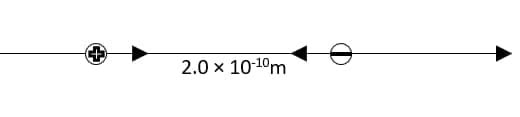enfurezca3x

2021-11-23

If an electron and a proton are expelled at the same time, $2.0×{10}^{-10}m$ apart (a typical atomic distance), determine each particle's starting acceleration.Louis Gregory

Step 1
Given
We are given the distance between a proton and an electron $r=2.0×{10}^{-10}m$. We are asked to calculate initial acceleration of each particle.
Solution
The two particles have an opposite charge, therefore each one exerts a force F on the other particle. This force could be calculated by the Coulomb's law as in equalion 21.2
$F=\frac{1}{4\pi {ϵ}_{0}}\frac{\mid {q}_{p}{q}_{e}\mid }{{r}^{2}}$
Where ${ϵ}_{0}$ is the electric constant and the term $\frac{1}{4\pi {ϵ}_{0}}$ equals $9.0×{10}^{9}N\cdot \frac{{m}^{2}}{{C}^{2}}$. ${q}_{p}$ is the charge of proton and equals $1.60×{10}^{-19}C$ and ${q}_{e}$, is the charge of electron and equals $1.60×{10}^{-19}C$ (See Appendix F)
To obtain the initial acceleration a of the two particles we should get the force that exerted on the proton and the electron. Where the acceleration a is given by Newton's second law in the form.
$a=\frac{F}{m}$
Where m is the mass of the particle, and for proton ${m}_{p}=1.67×{10}^{-19}kg$ while for electron ${m}_{e}=9.11×{10}^{-31}kg$ (See Appendix F)
Now we can plug our values tor ${g}_{p}$, ${g}_{e}$ and $\tau$ into equation (1) to get F
$F=\frac{1}{4\pi {ϵ}_{0}}\frac{\mid {q}_{p}{q}_{e}\mid }{{r}^{2}}$
$=\left(9.0×{10}^{9}N\cdot \frac{{m}^{2}}{{C}^{2}}\right)\frac{{\left(1.60×{10}^{-19}C\right)}^{2}}{{\left(2.0×{10}^{-10}m\right)}^{2}}$
$=5.76×{10}^{-9}N$
Step 2
Now, use equation (2) to get the acceleration a for each particle using the value of F.
For proton: We can plug our values for F and ${m}_{p}$ into equation (2) to get the initial acceleration a of the proton
${a}_{p}=\frac{F}{{m}_{p}}=\frac{5.76×{10}^{-9}N}{1.673×{10}^{-27}kg}=3.4×{10}^{18}\frac{m}{{s}^{2}}$
For electron Again plug our values for F and ${m}_{n}$, into equation (2) to gel the initial acceleration a of the electron
${a}_{e}=\frac{F}{{m}_{p}}=\frac{5.76×{10}^{-9}N}{9.109×{10}^{-31}kg}=6.3×{10}^{21}\frac{m}{{s}^{2}}$Ourst1977Proton
${m}_{p}=1.63×{10}^{-27}kg$
${q}_{p}=e=1.602×{10}^{-19}C$
Electron
${m}_{p}=9.109×{10}^{-31}kg$
${q}_{p}=-e=-1.602×{10}^{-19}C$
Since the charges are opposite, the Coulon b fuse is attractive.
$F=\frac{1}{4\pi {ϵ}_{0}}\frac{\mid {q}_{p}{q}_{e}\mid }{{r}^{2}}=\left(8.988×{10}^{9}\frac{N{m}^{2}}{{c}^{2}}\right)\frac{{\left(1.602×{10}^{-19}C\right)}^{2}}{{\left(2.0×{10}^{-10}m\right)}^{2}}=5.766709788×{10}^{-9}$
From Newtois Second Law, $F=ma⇒a=\frac{F}{m}$
$\therefore$ acceleration of Proton:
${a}_{p}=\frac{F}{{m}_{p}}=\frac{5.766709788×{10}^{-9}N}{1.673×{10}^{-27}kg}=3.4469×{10}^{18}\frac{m}{{v}^{2}}$
$\therefore {a}_{p}=3.4×{10}^{18}\frac{m}{{v}^{2}}$
acceleration of electron:
${a}_{e}=\frac{F}{{m}_{e}}=\frac{5.766709788×{10}^{-9}N}{9.109×{10}^{-31}kg}=6.33078×{10}^{27}\frac{m}{{v}^{2}}$
$\therefore {a}_{p}=6.3×{10}^{18}\frac{m}{{v}^{2}}$

Do you have a similar question?